# Topological product

(diff) ← Older revision | Latest revision (diff) | Newer revision → (diff)

Tikhonov product, of a family of topological spaces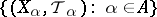The topological space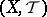whereis the Cartesian product (i.e. the complete direct product) of the setsover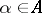and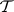is the weakest (i.e. the smallest) topology (cf. Topological structure (topology)) onsuch that all the natural projection mappings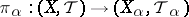are continuous. The topology, moreover, is called the product topology, while the Tikhonov productis also said to be the topological product of the family of spaces.

The standard base of the topological spaceis the family of all sets of the form, where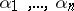is an arbitrary finite collection of elements of the index setand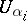is an arbitrary element of the topology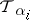,.

In particular, if the familyconsists of two spacesand, then a base of the topology of the product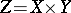is formed by sets of the form, whereis an arbitrary open set inandis an arbitrary open set in. A base of the topology of the product of an arbitrary finite ordered set of topological spaces can be described similarly. Examples of topological products are: the plane, which is the product of two straight lines; the-dimensional space, which is the product ofstraight lines; and a torus, which is the product of two circles.

The earliest attempts to define the topological product of an infinite set of topological spaces was for the case of metrizable factors. Accordingly, attempts were made to describe the product topology in terms of convergence of ordinary (countable) sequences. When the family of factors is uncountable, it proved impossible to obtain the above result in this way, because the closure operation in the topological product of an uncountable set of non-singleton metrizable spaces cannot be described in the language of convergence of sequences, nor can it be reduced to the closure of countable sets.

The definition of the topological product of an infinite set of topological spaces was given by A.N. Tikhonov (1930). He also proved that the topological product of compact Hausdorff spaces is always a compact Hausdorff space (Tikhonov's theorem).

The construction of a topological product is one of the main tools in the formation of new topological objects from ones already existing. Using topological products one can construct a number of fundamental standard objects of general topology, in particular, a Tikhonov cube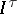, defined as the topological product of a family of cardinalityof intervals of the real line. By Tikhonov's theorem, all Tikhonov cubes are compact. Tikhonov proved that every completely-regular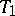-space is homeomorphic to a subspace of a cube.

In addition to the cubes, an important role is played in topology by the spaces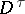and, which are the products ofcopies of spaces consisting, respectively, of two isolated points (the simple digon) and of digons with one isolated point (the connected digon). Every compact metric space is a continuous image of a Cantor perfect set, i.e. of a space homeomorphic to the product of a countable number of simple digons; every zero-dimensional space, i.e. every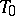-space in which the open-closed sets form a base, is homeomorphic to a subset of a Cantor discontinuum; every-space is homeomorphic to a subset of the space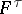.

Related to the power and role of the operation of taking topological products, problems concerned with the behaviour of topological properties under formation of topological products are of central importance in general topology. The classes of Hausdorff spaces, regular spaces and completely-regular spaces are stable under the operation of taking topological products. However, the product of a normal space with an interval need not be a normal space. Even for a finite number of factors, important topological properties such as the Lindelöf property and paracompactness are not stable under taking topological products.

The theorem that follows is of importance in general topology and its applications (particularly in the construction of models of set theory): The Suslin number of the topological product of an arbitrary set of separable topological spaces is countable. In particular, the Suslin number of an arbitrary Tikhonov cube is countable.

By distinguishing certain subspaces in a full topological product, the operation of taking topological products gives rise to the useful operations of the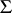-product and the-product. The operation of taking the box product of topologies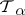leads to a completely-different topology on the Cartesian product of the sets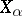.

How to Cite This Entry:
Topological product. Encyclopedia of Mathematics. URL: http://encyclopediaofmath.org/index.php?title=Topological_product&oldid=18295
This article was adapted from an original article by A.V. Arkhangel'skii, V.V. Fedorchuk (originator), which appeared in Encyclopedia of Mathematics - ISBN 1402006098. See original article